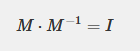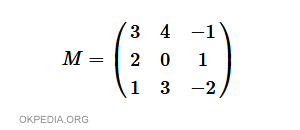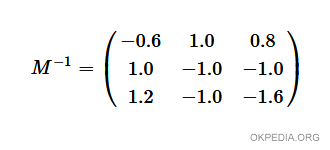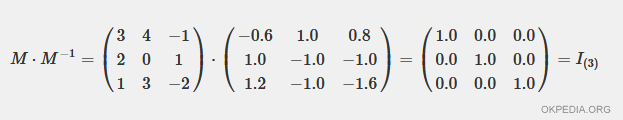# How to find an inverse matrix in Python

To calculate the inverse matrix in Python, use the linalg.inv() function of the numpy module.

linalg.inv(x)

The parameter x of the function is a square invertible matrix M defined with the function array() of numpy.

The function outputs the inverse matrix M-1 of the matrix M.

What is the inverse matrix? The inverse matrix M-1 of a square matrix M is another square matrix, such that the product M · M-1 is equal to an identity matrix I.## Example

Given the following invertible matrix M, find the inverse matrix M-1.Import the numpy module in Python

>>> import numpy as np

Now, define the input matrix using the array() function.

>>> m=np.array([[3,4,-1],[2,0,1],[1,3,-2]])

Calculate the inverse matrix with the function linalg.inv().

>>> np.linalg.inv(m)

The function calculates and outputs the inverse matrix

>>> array([[-0.6, 1. , 0.8],
[ 1. , -1. , -1. ],
[ 1.2, -1. , -1.6]])

The inverse output matrix is also an array() object. It can be read as a list.

The elements of the inverse matrix are real numbers.Verification. The product of the matrix M for the matrix M-1 is an identity matrix.https://how.okpedia.org/en/python/how-to-find-an-inverse-matrix-in-pythonReport us an error or send a suggestion to improve this page

Matrix in Python